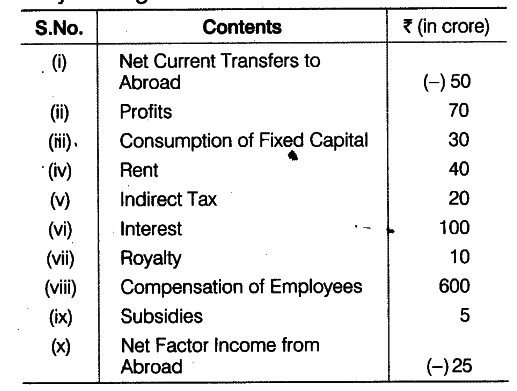# Calculate the Gross National Product at Market price

Calculate (a) Gross National Product at Market Price and (b) Net National Disposable Income from the following data:(a) Gross National Product at Market Price (\$GN{{P}_{MP}}\$ ) = Compensation of Employees + (Rent + Interest + Profit + Royalty)

• Net Factor Income from Abroad + Consumption of Fixed Capital
• Net Indirect Tax (Indirect Tax - Subsidies)
= 600 + (40 +100+70 +10) + (- 25)
• 30+ (20-5)
= 865 - 25 = Rs 840 crore
(b) Net National Disposable Income =
\$GN{{P}_{MP}}\$ - Consumption of Fixed Capital - Net Current Transfers to Abroad = 840-30-(-50)
= 840-30 + 50
= 860 crore